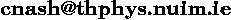# Charles Nash## Department of Mathematical Physics, National University of Ireland, Maynooth, Ireland.

tel: +353 1 708 3764 (direct line to office)
FAX: +353 1 708 3967Research interests: Quantum field theory and differential topology.
Research into relativistic quantum mechanical systems known as quantum field theories using both analytical and topological techniques.
It is a feature of the past twenty five years of research in quantum field theory that the way forward in many problems is considerably illuminated if, in addition to analysis, one uses topology.
The famous Yang--Mills equations, invented by theoretical physicists, have also been the source of many new results in mathematics these being mainly in three and four dimensional differential geometry and topology. Thus this is a rich field with many open problems and interconnections with other disciplines.
The analytical and topological aspects can be combined in a geometric study of the renormalisation group and this is also under study.
A key recent topic of mine is an investigation of quantum field theory models on the lattice in order to shed insight into suitable approximation methods which may be tractable for basic calculations. This has resulted so far in results relating the topology of the continuum quantum field situation with the lattice combinatorial one. This rather like the relation between the combinatorial formulation of cohomology and the continuum de Rham formulation using calculus (i.e. differential forms). There are interesting new, and calculable, features of the energy momentum tensors of such models and links with the modular invariance of string theory.
Recent papers and books